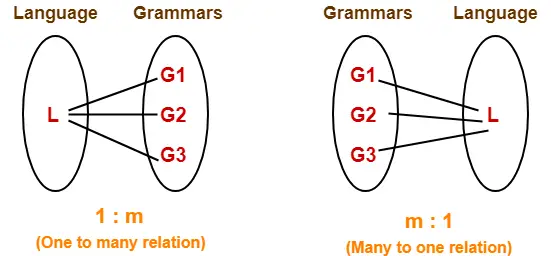# Language Of Grammar | Automata

## Language Of Grammar-

 Language of Grammar is the set of all strings that can be generated from that grammar.

• If the language consists of finite number of strings, then it is called as a Finite language.
• If the language consists of infinite number of strings, then it is called as an Infinite language.

### Example-01:

Consider a grammar G = (V , T , P , S) where-

• V = { S }
• T = { a , b }
• P = { S → aSbS , S → bSaS , S → ∈ }
• S = { S }

This grammar generates the strings having equal number of a’s and b’s.

So, Language of this grammar is-

 L(G) = { ∈ , ab , ba , aabb , bbaa , abab , baba , …… }

• This language consists of infinite number of strings.
• Therefore, language of the grammar is infinite.

### Example-02:

Consider a grammar G = (V , T , P , S) where-

• V = { S , A , B , C }
• T = { a , b , c }
• P = { S → ABC , A → a , B → b , C → c }
• S = { S }

This grammar generates only one string “abc”.

So, Language of this grammar is-

 L(G) = { abc }

• This language consists of finite number of strings.
• Therefore, language of the grammar is finite.

Also Read- Deciding Language Is Finite Or Infinite

## Important Concept-

• For any given grammar, the language generated by it is always unique.
• For any given language, we may have more than one grammar generating that language.## Example-

Consider the following two grammars-

### Grammar G1-

S → AB

A → a

B → b

The language generated by this grammar is-

L(G1) = { ab }

### Grammar G2-

S → AB

A →

B → ab

The language generated by this grammar is-

L(G2) = { ab }

Here,

• Both the grammars generate a unique language.
• But given a language L(G) = { ab }, we have two different grammars generating that language.

This justifies the above concept.

Next Article- Language Ambiguity

Get more notes and other study material of Theory of Automata and Computation.

Watch video lectures by visiting our YouTube channel LearnVidFun.

SummaryArticle Name
Language Of Grammar | Automata
Description
In Automata, Language of Grammar is the set of all strings that can be generated from that grammar. Examples. Finite and Infinite Language- If language consists of finite number of strings, then it is called as a finite language otherwise an infinite language.
Author
Publisher Name
Gate Vidyalay
Publisher Logo